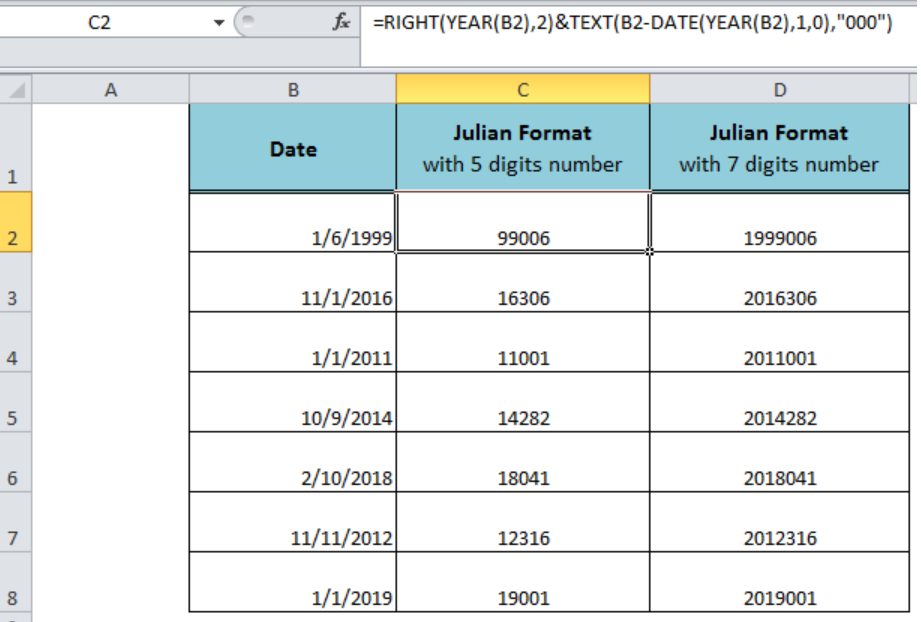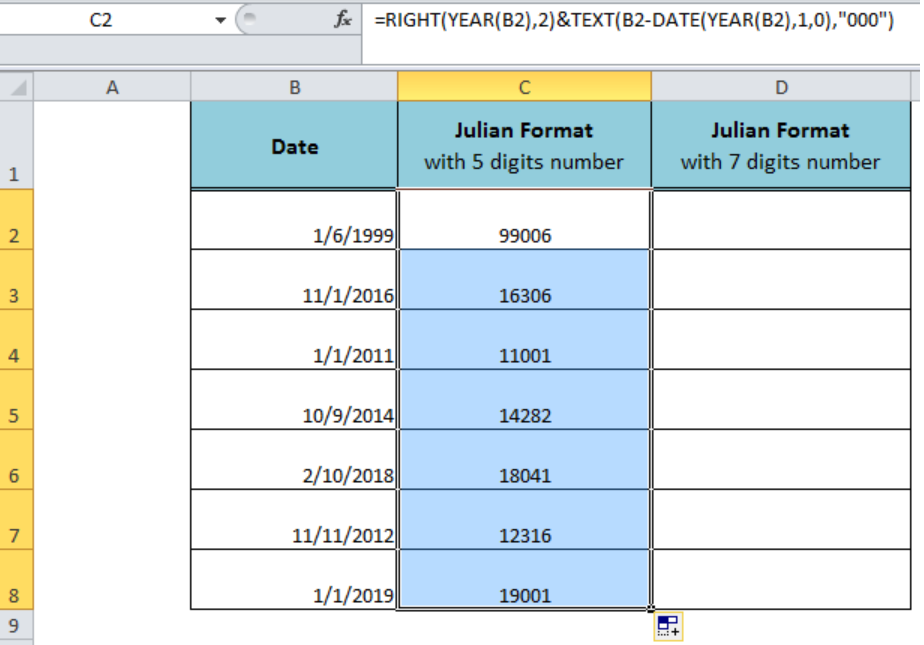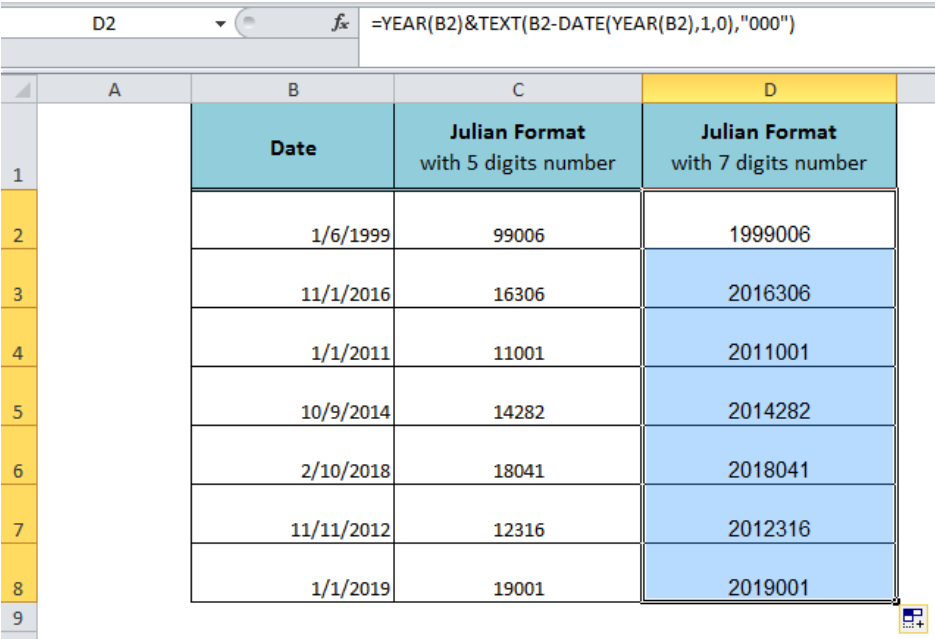Get instant live expert help with Excel or Google Sheets“My Excelchat expert helped me in less than 20 minutes, saving me what would have been 5 hours of work!”

#### Post your problem and you'll get expert help in seconds

Your message must be at least 40 characters
Our professional experts are available now. Your privacy is guaranteed.

# Convert date to Julian format

The Julian date format is generally used as a timestamp in programming, manufacturing or other related fields like a reference for a batch number. Generally, it has two format variations, comprising of 5 or 7 digits numbers, but in most of the cases, 5 digits format is used. Here, we will learn how to convert a date to Julian format in both of these formats.Figure 1. Converting a Date to Julian Format

## Converting a Date to 5 Digits Julian Format

Mostly 5 digits number of Julian formats is used where the first 2 digits represent the year and the last 3 digits represent the day of the year. In Excel, to convert a date to Julian format with 5 digits number, we need to use the following formula in cell C2 as per our example;

`=RIGHT(YEAR(B2),2)&TEXT(B2-DATE(YEAR(B2),1,0),"000")`

The first part of the formula, `RIGHT(YEAR(B2),2)`, extracts the first 2 digits representing the year, and the second part of the formula, `TEXT(B2-DATE(YEAR(B2),1,0), "000")`, returns the last 3 digits number representing the day of the year from a given date. Copy or drag down the fill handle to convert remaining dates to 5 digits Julian format.Figure 2. Converting a Date to 5 Digits Julian Format

## Converting a Date to 7 Digits Julian Format

In 7 digits Julian format, the first 4 digits represent the year and the last 3 digits represent the day of the year. In our example, to covert a date to Julian format with 7 digits number, we need to use the following formula in cell D2 and copy the formula to other dates;

`=YEAR(B2)&TEXT(B2-DATE(YEAR(B2),1,0),"000")`

The first part of the formula, `YEAR(B2)`, returns the 4 digits indicating the year and last part of the formula, `TEXT(B2-DATE(YEAR(B2),1,0), "000")`, returns the last 3 digits of the 7 digits Julian format.Figure 3. Converting a Date to 7 Digits Julian Format

## Instant Connection to an Expert through our Excelchat Service

Most of the time, the problem you will need to solve will be more complex than a simple application of a formula or function. If you want to save hours of research and frustration, try our live Excelchat service! Our Excel Experts are available 24/7 to answer any Excel question you may have. We guarantee a connection within 30 seconds and a customized solution within 20 minutes.

### Did this post not answer your question? Get a solution from connecting with the expert.Another blog reader asked this question today on Excelchat:## Subscribe to Excelchat.coAnother blog reader asked this question today on Excelchat: